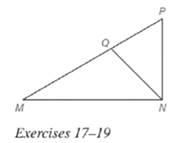Chapter 5.6, Problem 18E### Elementary Geometry for College St...

6th Edition
Daniel C. Alexander + 1 other
ISBN: 9781285195698

#### Solutions

Chapter
Section### Elementary Geometry for College St...

6th Edition
Daniel C. Alexander + 1 other
ISBN: 9781285195698
Textbook Problem
3 views

# Exercises 18 and 19 are based on a theorem ( not stated) that is the converse of Theorem 5.6.3. See the figure on above. Given: N P = 4 , M N = 8 , P Q = 3 , and M Q = 6 ; m ∠ P = 63 ∘ and m ∠ M = 27 ∘ Find: m ∠ P N Q (HINT: N P M N = P Q M Q . )To determine

To find:

mPNQ.

Explanation

Given:

NQ bisects MNP, NP=4,MN=8,PQ=3,MQ=6,mP=63,mM=27.

Theorem used:

Angle bisector theorem:

If a ray bisects one angle of a triangle, then it divides the opposite side into segments whose lengths are proportional to the lengths of the two sides that form the bisected angle.

Calculation:

Given: NQ bisects MNP.

Then by angle-bisector theorem, the ray NQ divides the opposite side into segments whose lengths are proportional to the lengths of the two sides that form the bisected angle.

NPMN=PQMQ

In a triangle the sum of the angles is 180.

### Still sussing out bartleby?

Check out a sample textbook solution.

See a sample solution

#### The Solution to Your Study Problems

Bartleby provides explanations to thousands of textbook problems written by our experts, many with advanced degrees!

Get Started

#### Find more solutions based on key concepts